# 引言

​ 本文讲述霍夫变换的一些内容，并加入一些理解性东西，参考了部分博客等相关性内容。希望能对霍夫变换有所了解，也希望看到的人如果发现错误及时帮忙纠正。博主水平有限，还望赐教。

# 历史和简介

## 历史

​霍夫变换（Hough Transform）是在1959年由气泡室（Bubble Chamber）照片的机器分析而发明，发明者Paul Hough在1962年获得美国专利，被命名为Method and Means for Recognizing Complex Patterns（用于识别复杂图案的方法和手段）。该专利对直线采用斜截距参数化，但由于斜率可能变成无穷大，这有可能导致无限变换空间（unbounded transform space）。

​现在使用的霍夫变换是1972年由Richard Duda和Peter Hart所发明，称为“广义霍夫变换[GHT]”（Use of the Hough Transformation to Detect Lines and Curves in Pictures，1972）。

​然后1981年在Dana H. Ballard的计算机视觉社区中出现一篇文章名为 Generalizing the Hough transform to detect arbitrary shapes，从而推广开来。

​该文描述了使用模板匹配原理对霍夫变换进行修改。要知道霍夫变换最初是为了分析定义的形状（如线、圆、椭圆等）而开发。通过了解其形状并旨在其找出图像中的位置和方向，这种改变使得霍夫变换能够检测用其模型描述的任意对象。这将图像中查找对象（用模型描述）的问题通过查找模型在图像中的位置来解决。利用广义霍夫变换（GHT），找到模型位置的问题转换为寻找将模型映射到图像中的变换参数的问题。给定变换参数的值，就可以确定模型在图像中的位置。

​后来产生了更多霍夫变换的变体和扩展，比如KHT,3DKHT，这里不细致说明。

## 简介

​霍夫变换是一个特征提取技术。其可用于隔离图像中特定形状的特征的技术，应用在图像分析、计算机视觉和数字图像处理领域。目的是通过投票程序在特定类型的形状内找到对象的不完美实例。这个投票程序是在一个参数空间中进行的，在这个参数空间中，候选对象被当作所谓的累加器空间中的局部最大值来获得，所述累加器空间由用于计算霍夫变换的算法明确地构建。最基本的霍夫变换是从黑白图像中检测直线(线段)。Hough变换主要优点是能容忍特征边界描述中的间隙，并且相对不受图像噪声的影响。

# 原理

​霍夫变换最简单的是检测直线。我们知道，直线的方程表示可以由斜率和截距表示（这种表示方法，称为斜截式），如下所示：

y=mx+b(1) (1) y = m x + b

如果用参数空间表示则为 (b,m) ( b , m ) $(b,m)$,即用斜率和截距就能表示一条直线。

但是这样会参数问题，垂直线的斜率不存在（或无限大），这使得斜率参数 m m $m$的值接近于无限。为此，为了更好的计算，Richard O. Duda和Peter E. Hart在1971年4月，提出了Hesse normal form(Hesse法线式)

$\begin{array}{}\text{(2)}& r=x\mathrm{cos}\theta +y\mathrm{sin}\theta \end{array}$

其中 r r $r$是原点到直线上最近点的距离(其他人可能把这记录为$\rho$$\rho$，下面也可以把 r r $r$看成参数$\rho$$\rho$)， θ θ $\theta$ x x $x$轴与连接原点和最近点直线之间的夹角。如图1所示。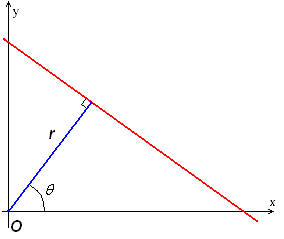因此，可以将图像的每一条直线与一对参数$\left(r,\theta \right)$$(r,\theta)$相关联。这个参数 (r,θ) ( r , θ ) $(r,\theta)$平面有时被称为霍夫空间，用于二维直线的集合。

经过Hough变换，将图像空间中的一个点映射到Hough空间，如图2所示。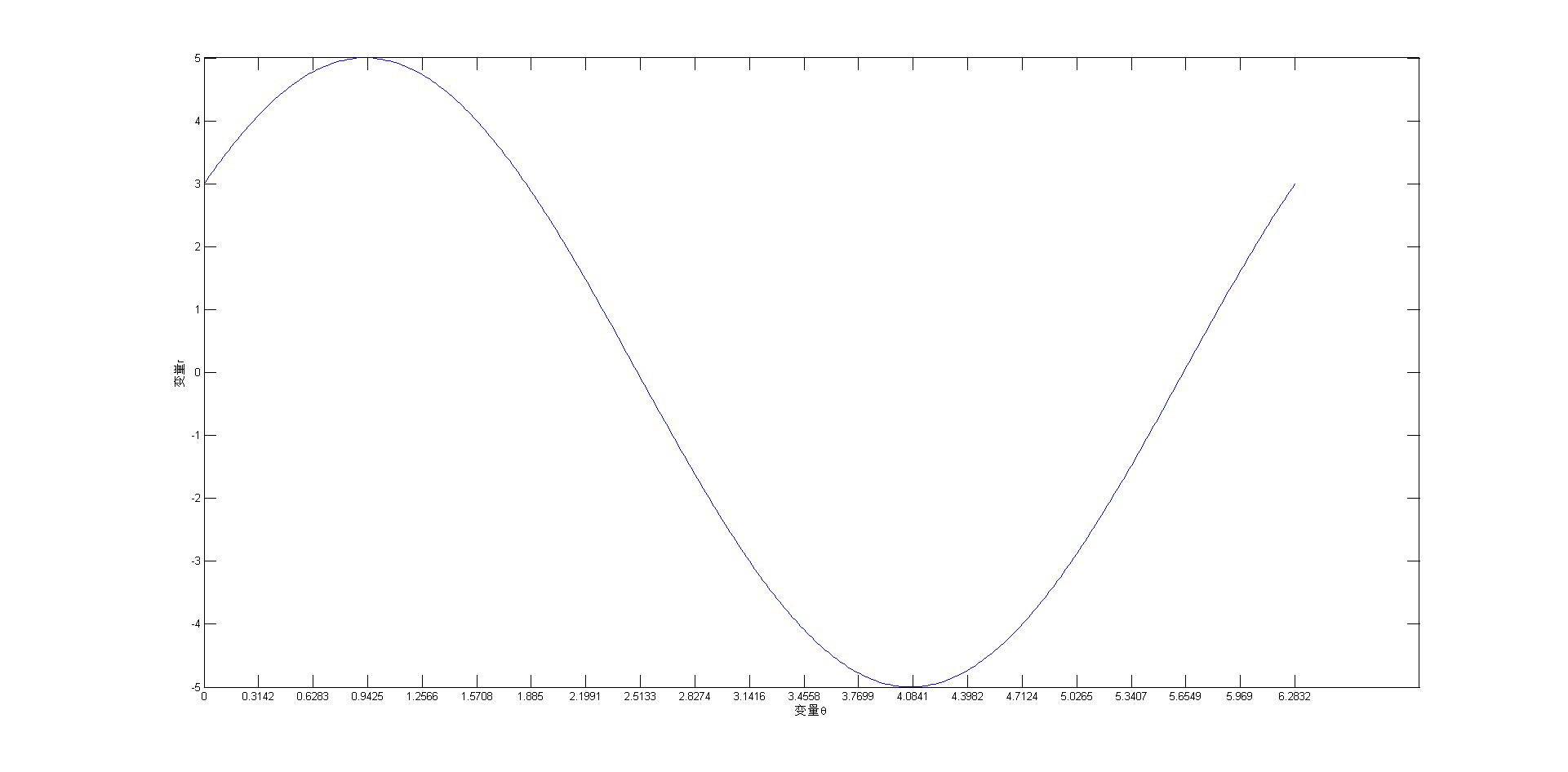% 一个点的坐标为（3,4）
x=3;
y=4;
%将给定的一个定点映射到霍夫变换空间
theta=0:pi/200:2*pi;% 角度
r=x*cos(theta)+y*sin(theta);
plot(theta,r);%绘图
set(gca,'XTick',[0:pi/10:2*pi]);   % 修改x轴坐标间隔
xlabel('变量\theta')
ylabel('变量r')

继续正题内容，图2显示了经过定点（3,4）时$\left(r,\theta \right)$$(r,\theta)$的关系。显示了在极坐标对极径极角平面绘出所有通过该定点的直线, 将得到一条正弦曲线。正弦曲线的形状取决于，点到所定义原点的距离 r r $r$。通常，$r$$r$越大，正弦曲线的振幅越大，反之则会越小。

所以我们可以得到一个结论，给定平面中的单个点，那么通过该点的所有直线的集合对应于 (r,θ) ( r , θ ) $(r,\theta)$平面中的正弦曲线，这对于该点是独特的。一组两个或更多点形成一条直线将产生在该线的 (r,θ) ( r , θ ) $(r,\theta)$处交叉的正弦曲线。因此，检测共线点的问题可以转化为找到并发曲线的问题。

## 例子1

考虑下面三个点，这里显示为黑点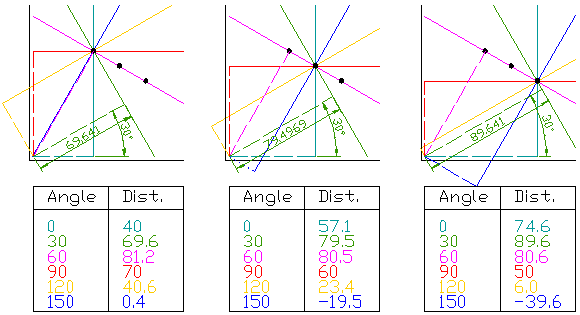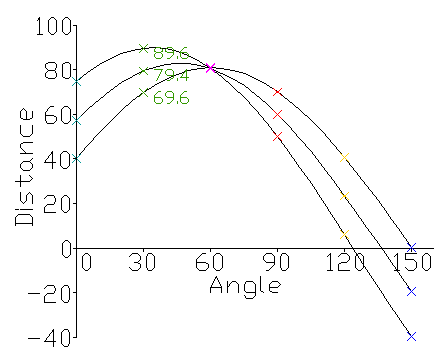图4显示的曲线相交的点给出距离和角度。该距离和角度表示与被测试点相交的线。在图4中，所示的线条在粉红点相交; 这对应于图3中的实线粉红线，其穿过所有三个点。

在图像分析上下文，边缘段的点（一个或多个）的坐标 (xi,yi) ( x i , y i ) $(x_i,y_i)$在图像中是已知的，并且因此作为参数线等式中的常量，而 r r $r$$\theta$$\theta$是未知变量是我们要寻找的。如果我们绘制由 (r,θ) ( r , θ ) $(r,\theta)$每个定义的可能值 (xi,yi) ( x i , y i ) $(x_i,y_i)$，笛卡尔图像空间中的点映射到极性霍夫参数空间中的曲线（即正弦曲线）。这个点到曲线的变换是直线的霍夫变换。当在霍夫参数空间中查看时，在笛卡尔图像空间中共线的点变得很明显，因为它们产生在相同 (r,θ) ( r , θ ) $(r,\theta)$点相交的曲线。

## 例子2

以下是显示包含两条粗线的光栅图像上的霍夫变换结果的不同示例。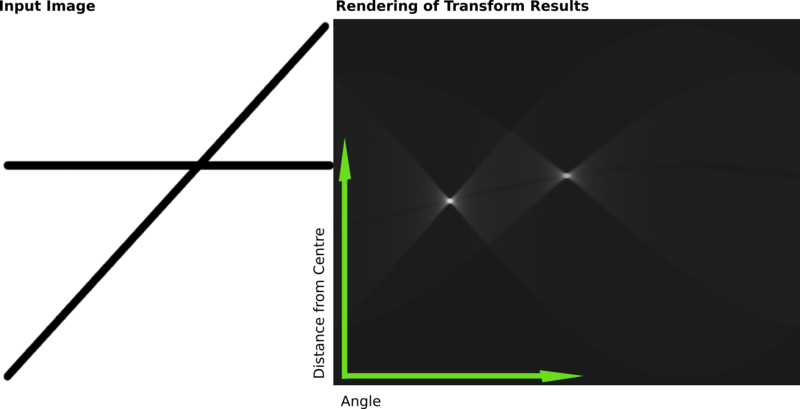该变换的结果存储在矩阵中。单元格值表示通过任意点的曲线数量。更高的单元格值变得更亮。两个明显的亮点是两条线的霍夫参数。从这些点的位置，可以确定输入图像中两条线的图像中心的角度和距离。

# 霍夫变换提取直线如何实现？实现机理是为何？

通过将霍夫参数空间量化为有限间隔或累加器单元来实现变换。随着算法的运行，每个算法都把 (xi,yi) ( x i , y i ) $(x_i,y_i)$转换为一个离散化的 (r,θ) ( r , θ ) $(r,\theta)$曲线，并且沿着这条曲线的累加器单元被递增。累加器阵列中产生的峰值表示图像中存在相应的直线的有力证据。
注意，现在我们考虑的是直线的霍夫变换（先不去考虑圆，圆的情况稍后简单说明）。累加器阵列的维度是二维的（也就是 r r $r$$\theta$$\theta$)。

那么对于图像来说， (x,y) ( x , y ) $(x,y)$处的每个像素及其邻域，霍夫变换算法被用于确定该像素是否有足够的直线证据。如果是，它将计算该线的参数 (r,θ) ( r , θ ) $(r,\theta)$，然后查找参数落入的累加器箱，并增加该箱的值（投票值）。通过查找具有最高值的箱，通常通过查找累加器空间中的局部最大值，可以提取最可能的线，并且读出它们的（近似的）几何定义。

找到这些峰的最简单方法是通过应用某种形式的阈值，但其他技术可能在不同情况下产生更好的结果 - 确定找到哪些行以及有多少。由于返回的行不包含任何长度信息，因此通常有必要在下一步中查找图像的哪些部分与哪些行匹配。此外，由于边缘检测步骤中存在缺陷误差，通常会在累加器空间中出现错误，这可能使得找到合适的峰值以及适当的线条变得非常重要。

线性霍夫变换的最终结果是类似于累加器的二维阵列（矩阵），该矩阵的一个维度是量化角度 θ θ $\theta$，另一个维度是量化距离 r r $r$。矩阵的每个元素的值等于位于由量化参数 $\left(r,\theta \right)$$(r,\theta)$表示的线上的点或像素的总和。所以具有最高值的元素表示输入图像中代表最多的直线。

在某些论文中，可能把累计器单元的结果认为是投票值。换句话说，将每个交点看成一次投票，也就是说 A(r,θ)=A(r,θ)+1 A ( r , θ ) = A ( r , θ ) + 1 $A(r,\theta)=A(r,\theta)+1$，所有点都如此进行计算后，可以设置一个阈值，投票大于这个阈值的可以认为是找到的直线。下图是从一个博客摘用。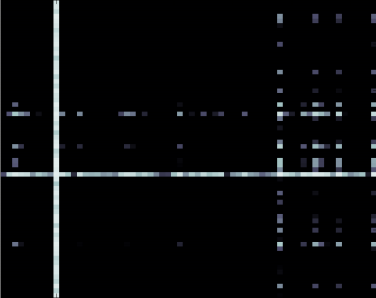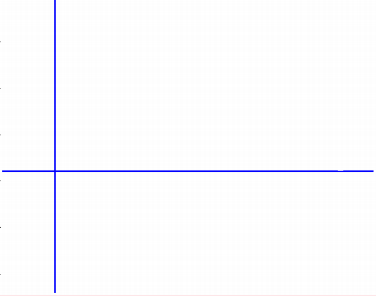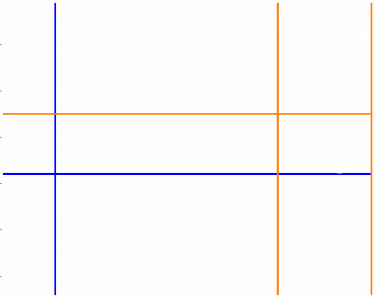对于大于阈值的点,有其Hough space的参数对 (r,θ) ( r , θ ) $(r,\theta)$ 通过逆映射我们可以得到图像空间中的直线：

y=cos(θl)sin(θl)x+ρlsin(θl)(3) (3) y = − c o s ( θ l ) s i n ( θ l ) x + ρ l s i n ( θ l )

# 实现使用的例子说明描述

霍夫变换可用于识别最适合一组给定边缘点的曲线的参数。该边缘描述通常从诸如Roberts Cross，Sobel或 Canny边缘检测器的特征检测算子获得，并且可能是嘈杂的，即其可能包含对应于单个整体特征的多个边缘片段。此外，由于边缘检测器的输出仅限定图像中的特征的位置，所以霍夫变换进一步是确定两个特征是什么（即检测其具有参数（或其他）的特征描述）以及 它们有多少个存在于图像中。

为了详细说明霍夫变换，我们从两个闭合矩形的简单图像开始。

## 简单闭合矩形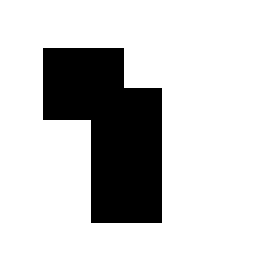使用 Canny边缘检测器可产生一组边界描述的这个部分，如图8所示。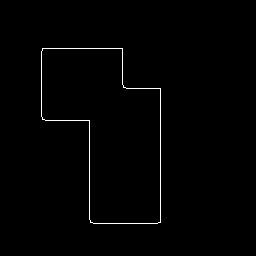这里我们看到了图像中的整体边界，但是这个结果并没有告诉我们这个边界描述中的特征的身份（和数量）。在这种情况下，我们可以使用Hough（线检测）变换来检测该图像的八个单独的直线段，从而确定对象的真实几何结构。

如果我们使用这些边缘/边界点作为Hough变换的输入，则会 (r,θ) ( r , θ ) $(r,\theta)$ 在笛卡尔空间中的每个边缘点的极坐标空间中生成一条曲线。当被视为强度图像时，累加器阵列看起来像图9所示可以利用直方图均衡技术使得图像可以让我们看到包含在低强度像素值中的信息模式，如图10所示。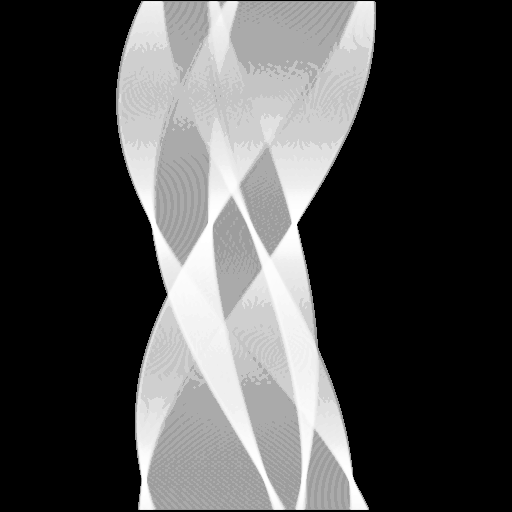注意，虽然 r r ​ $r​$ θ θ ​ $\theta​$是概念上的极坐标，但是累加器空间以横坐标 θ θ ​ $\theta​$和纵坐标 r r ​ $r​$的矩形绘制 。请注意，累加器空间环绕图像的垂直边缘，因此实际上只有8个实际峰值。

由梯度图像中的共线点生成的曲线 (r,θ) ( r , θ ) $(r,\theta)$ 在霍夫变换空间中的峰中相交。这些交点表征原始图像的直线段。有许多方法可用于从累加器阵列中提取这些亮点或局部最大值。例如，一个简单的方法涉及阈值处理，然后 对累加器阵列图像中孤立的亮点集群进行一些细化处理。这里我们使用相对阈值来提取 (r,θ) ( r , θ ) $(r,\theta)$ ，对应于原始图像中的每条直线边缘的点。（换句话说，我们只采用累加器数组中的那些局部最大值，其值等于或大于全局最大值的某个固定百分比。）

从Hough变换空间（即，反霍夫变换）映射回笛卡尔空间产生一组图像主题的线描述。通过将该图像叠加在原始的反转版本上，我们可以确认霍夫变换找到两个矩形的8个真实边的结果，并且因此揭示了遮挡场景的基础几何形状。

从Hough变换空间（即，反霍夫变换）映射回笛卡尔空间产生一组图像主题的线描述。通过将该图像叠加在原始的反转版本上（见图11），我们可以确认霍夫变换找到两个矩形的8个真实边的结果，并且因此揭示了遮挡场景的基础几何形状。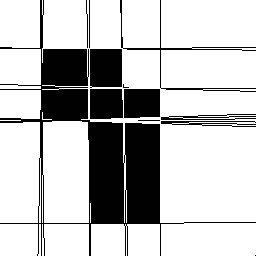请注意，在这个简单的例子中，检测到的和原始图像线的对齐精度显然不完美，这取决于累加器阵列的量化。（还要注意许多图像边缘有几条检测线，这是因为有几个附近的霍夫空间峰值具有相似的线参数值，存在控制这种效应的技术，但这里没有用来说明标准霍夫变换。）

还要注意，由霍夫变换产生的线条的长度是无限的。如果我们希望识别产生变换参数的实际线段，则需要进一步的图像分析以便查看这些无限长线的哪些部分实际上具有点。

为了说明Hough技术对噪声的鲁棒性，Canny边缘描述已被破坏1％（由椒盐噪声引起）， 在Hough变换之前，如图12所示。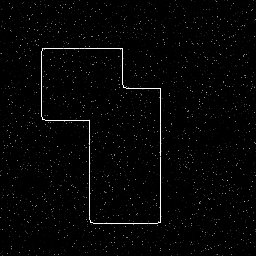绘制在霍夫空间的结果如图13所示。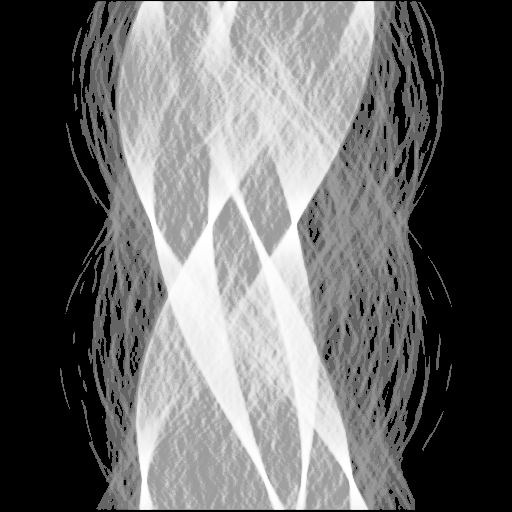将这个结果反霍夫变换（并将它覆盖在原来的结果上，见图14）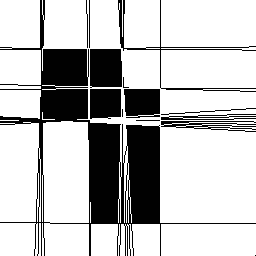可以通过变换图像来研究Hough变换对特征边界中的间隙的敏感性（使用了画图工具进行编辑，见图15）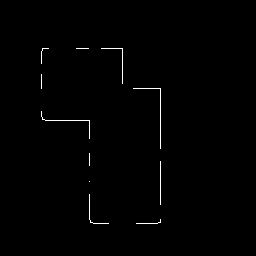然后我们再将其变换到霍夫变换空间，表示为图16所示。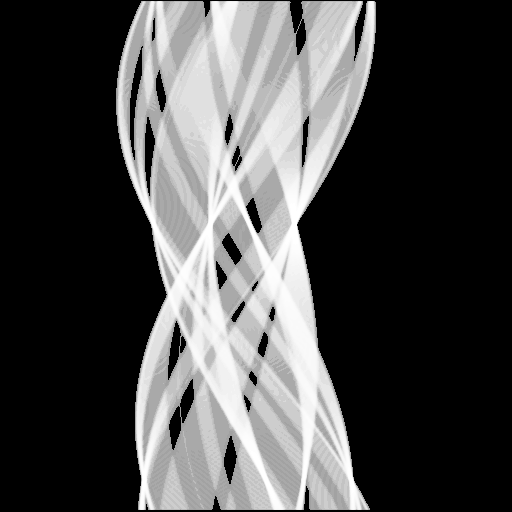然后使用40%的相对阈值去对图像反霍夫变换（同样也是叠加在原图上）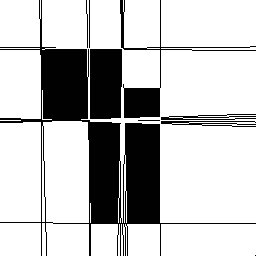在这种情况下，因为累加器空间没有接收到前面例子中的条目数量，只能找到7个峰值，但这些都是结构相关的线。

前面的例子都不是自然实例。下面展示自然实例的例子。

## 城市场景

在第一种情况下，我们有一个城市场景，这些建筑物被雾遮住，见图18。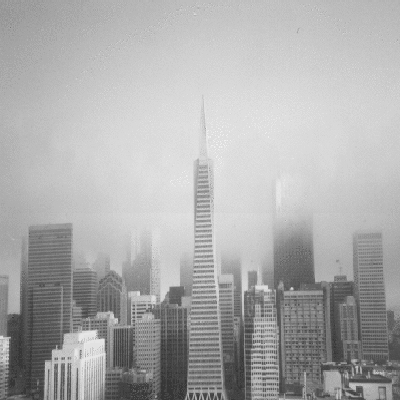如果我们想要找到建筑物的真实边缘，边缘检测器（例如Canny）无法很好地恢复这些信息，如图19所示。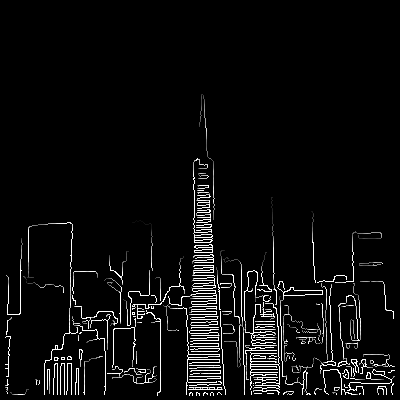但是，霍夫变换可以检测到一些表示遮挡区域内建筑物边缘的直线。原始图像的直方图均衡累加器空间表示如图20所示。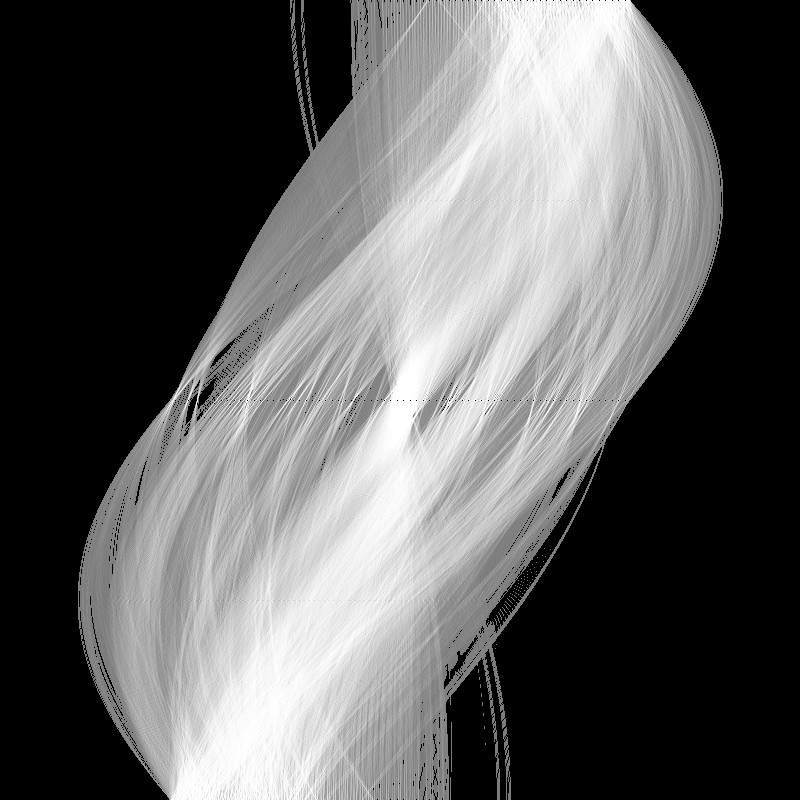如果我们将相对阈值设置为70％，我们会得到如图21所示的反霍夫变换图像。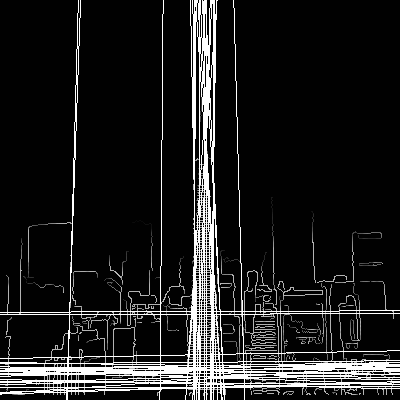这里只能检测到几条长边，并且在很多线条或边缘片段几乎共线的地方存在很多重复。应用更大的相对阈值（即50％）会产生如图22所示效果。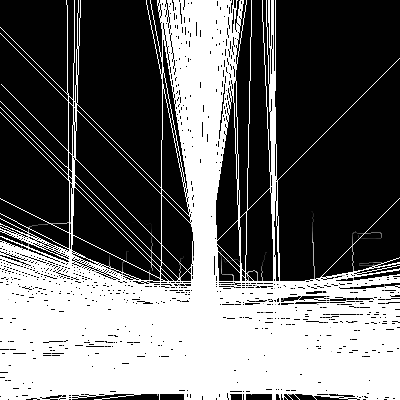会产生更多的预期线条，但会以许多共线边缘碎片产生的许多虚假线条为代价。

最后一个例子来自遥感应用。在这里，我们想要检测图像中的街道

## 遥感应用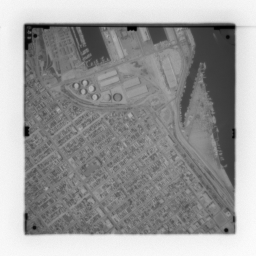图23显示了一个合理的矩形城市扇区（rectangular city sector）。我们可以使用Canny边缘检测器来检测该图像，如图24所示。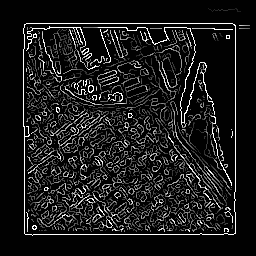但是，街道信息不能单独用作边缘检测器的输出。

图25表明霍夫线检测器能够恢复这些信息中的一些。但由于原始图像的对比度较差，因此确定了一组有限的特征（即街道）。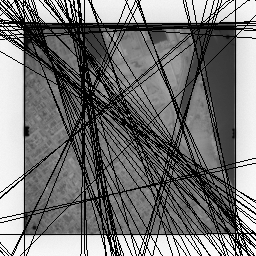# 实现算法描述

摘取一篇博客的算法描述：

1. 初始化 (θ,ρ) ( θ , ρ ) $(\theta,\rho)$空间， N(θ,ρ)=0 N ( θ , ρ ) = 0 $N(\theta,\rho)=0$ (N(θ,ρ)=0 ( N ( θ , ρ ) = 0 $(N(\theta,\rho)=0$ 表示在该参数表示的直线上的像素点的个数 ) ) $)$
2. 对于每一个像素点$\left(x,y\right)$$(x,y)$，在参数空间中找出满足 xcosθ+ysinθ=ρ x cos ⁡ θ + y sin ⁡ θ = ρ $x\cos\theta+y\sin\theta=\rho$ (θ,ρ) ( θ , ρ ) $(\theta,\rho)$对，然后令 (N(θ,ρ)=Nθ,ρ+1 ( N ( θ , ρ ) = N （ θ , ρ ） + 1 $(N(\theta,\rho)=N（\theta,\rho）+1$
3. 统计所有 N(θ,ρ) N ( θ , ρ ) $N(\theta,\rho)$的大小，取出 N(θ,ρ)>τ N ( θ , ρ ) > τ $N(\theta,\rho)>\tau$的参数 （ τ τ $\tau$是预设的阈值）

但我觉得这并不是十分完整的算法流程。所以我将其改进描述如下

1. 读取原始图并转换成灰度图，采用边缘检测算子（如Canny）转换成二值化边缘图像
2. 然后对该图像进行霍夫变换
3. 先使用峰值检测函数，找到大于阈值的霍夫变换单元（局部最大值应该最可能是线，步长和量化会影响效果）
4. 将上述识别出的一组候选峰，需要确定与其相关的线段及其起始点和终止点（这需要一定的算法，很多论文对此都做了改进，诸如蝴蝶形状宽度，峰值走廊）
5. 然后描绘于原图（或结果图）上

# 代码实现

## matlab版本

原图%读取图像
% 转换成灰度图
grayI = rgb2gray(I);

% 创建二进制图像
BW = edge(grayI,'canny');
% 使用二值图像创建Hough变换。
[H,T,R] = hough(BW);
imshow(H,[],'XData',T,'YData',R,...
'InitialMagnification','fit');
xlabel('\theta'), ylabel('\rho');
axis on, axis normal, hold on;
% 在图像的霍夫变换中查找峰值
P  = houghpeaks(H,5,'threshold',ceil(0.3*max(H(:))));
x = T(P(:,2)); y = R(P(:,1));
plot(x,y,'s','color','white');
% 找到线条并绘制它们
lines = houghlines(BW,T,R,P,'FillGap',5,'MinLength',7);
figure, imshow(I), hold on
max_len = 0;
for k = 1:length(lines)
xy = [lines(k).point1; lines(k).point2];
plot(xy(:,1),xy(:,2),'LineWidth',2,'Color','green');

% 绘制线条的开始和结束
plot(xy(1,1),xy(1,2),'x','LineWidth',2,'Color','yellow');
plot(xy(2,1),xy(2,2),'x','LineWidth',2,'Color','red');

% 确定最长线段的端点
len = norm(lines(k).point1 - lines(k).point2);
if ( len > max_len)
max_len = len;
xy_long = xy;
end
end
% 通过为青色着色来突出显示最长的线段
plot(xy_long(:,1),xy_long(:,2),'LineWidth',2,'Color','cyan');

运行结果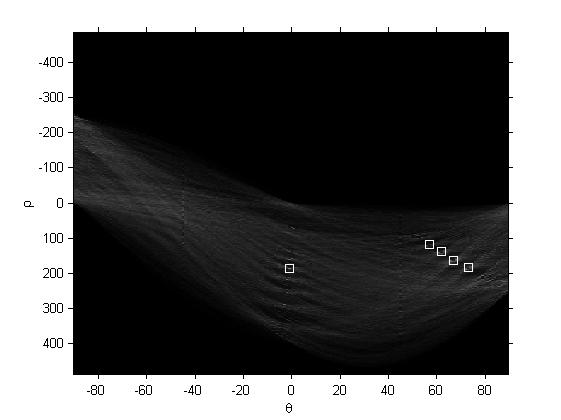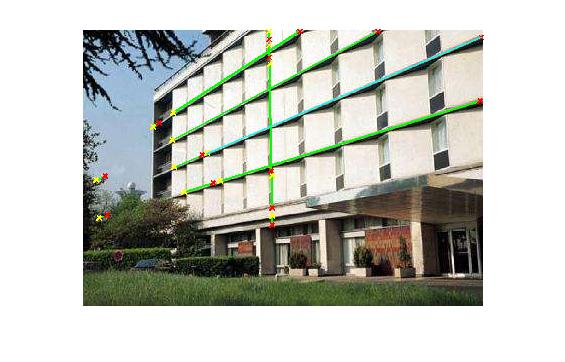只是个示范使用，参数可自调。

## python实现

由于本博主电脑是python2,python3共存，所以需要加入一定的注释表明是用哪个版本的python。要运行下面代码需要安装几个东西，在cmd命令行进行运行。代码本人是用python2编辑。（如果只装一个版本，下面的安装代码请自行调整），我没有将其逆霍夫变换回原图上了，大家可以自行绘制。

py -2 -m pip install numpy
py -2 -m pip install matplotlib
py -2 -m pip install scipy
py -2 -m pip install scikit-image

源码

#! python2
# coding: utf-8

import numpy as np
import matplotlib.pyplot as plt

from skimage.transform import hough_line
from skimage.draw import line

img = np.zeros((100, 150), dtype=bool)
img[30, :] = 1
img[:, 65] = 1
img[35:45, 35:50] = 1
rr, cc = line(60, 130, 80, 10)
img[rr, cc] = 1
img += np.random.random(img.shape) > 0.95

out, angles, d = hough_line(img)

fix, axes = plt.subplots(1, 2, figsize=(7, 4))

axes.imshow(img, cmap=plt.cm.gray)
axes.set_title('Input image')

axes.imshow(
out, cmap=plt.cm.bone,
axes.set_title('Hough transform')
axes.set_xlabel('Angle (degree)')
axes.set_ylabel('Distance (pixel)')

plt.tight_layout()
plt.show()


运行结果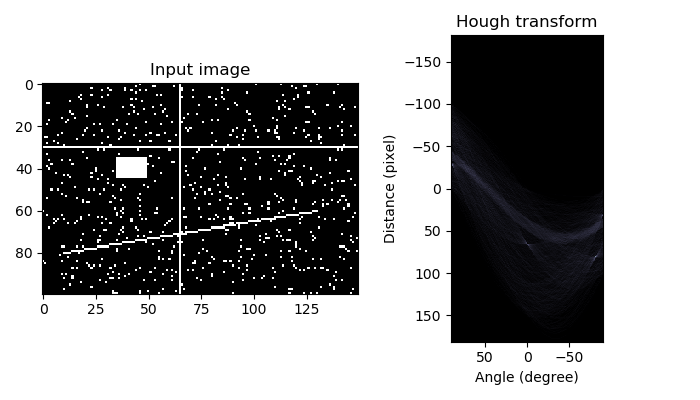## Opencv实现

opencv的关于霍夫变换提取的函数可以在Opencv的该文档见到
Opencv关于houghlines函数
博主电脑安装的是opencv2.4.13版本，代码是来自于浅墨大神（毛星云）的代码实现。

实验的原图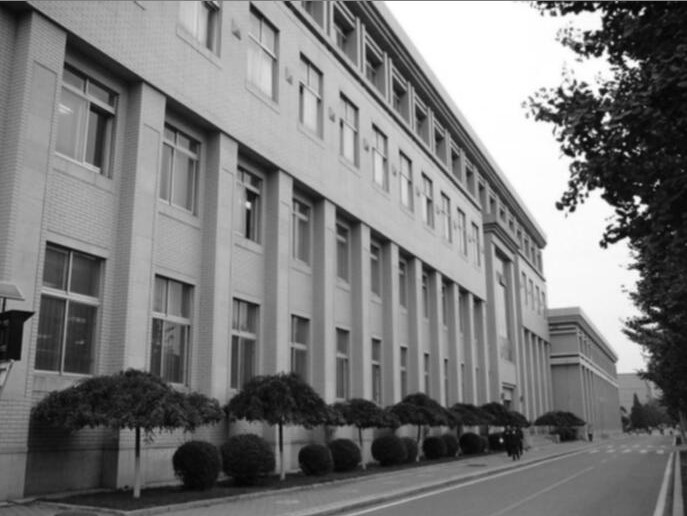//-----------------------------------【头文件包含部分】---------------------------------------
//      描述：包含程序所依赖的头文件
//----------------------------------------------------------------------------------------------
#include <opencv2/opencv.hpp>
#include <opencv2/imgproc/imgproc.hpp>

//-----------------------------------【命名空间声明部分】---------------------------------------
//      描述：包含程序所使用的命名空间
//-----------------------------------------------------------------------------------------------
using namespace cv;
//-----------------------------------【main( )函数】--------------------------------------------
//      描述：控制台应用程序的入口函数，我们的程序从这里开始
//-----------------------------------------------------------------------------------------------
int main()
{
//【1】载入原始图和Mat变量定义
Mat midImage, dstImage;//临时变量和目标图的定义

//【2】进行边缘检测和转化为灰度图
Canny(srcImage, midImage, 50, 200, 3);//进行一此canny边缘检测
cvtColor(midImage, dstImage, CV_GRAY2BGR);//转化边缘检测后的图为灰度图

//【3】进行霍夫线变换
vector<Vec2f> lines;//定义一个矢量结构lines用于存放得到的线段矢量集合
HoughLines(midImage, lines, 1, CV_PI / 180, 150, 0, 0);

//【4】依次在图中绘制出每条线段
for (size_t i = 0; i < lines.size(); i++)
{
float rho = lines[i], theta = lines[i];
Point pt1, pt2;
double a = cos(theta), b = sin(theta);
double x0 = a*rho, y0 = b*rho;
pt1.x = cvRound(x0 + 1000 * (-b));
pt1.y = cvRound(y0 + 1000 * (a));
pt2.x = cvRound(x0 - 1000 * (-b));
pt2.y = cvRound(y0 - 1000 * (a));
line(dstImage, pt1, pt2, Scalar(55, 100, 195), 1, CV_AA);
}

//【5】显示原始图
imshow("【原始图】", srcImage);

//【6】边缘检测后的图
imshow("【边缘检测后的图】", midImage);

//【7】显示效果图
imshow("【效果图】", dstImage);

waitKey(0);

return 0;
}

运行结果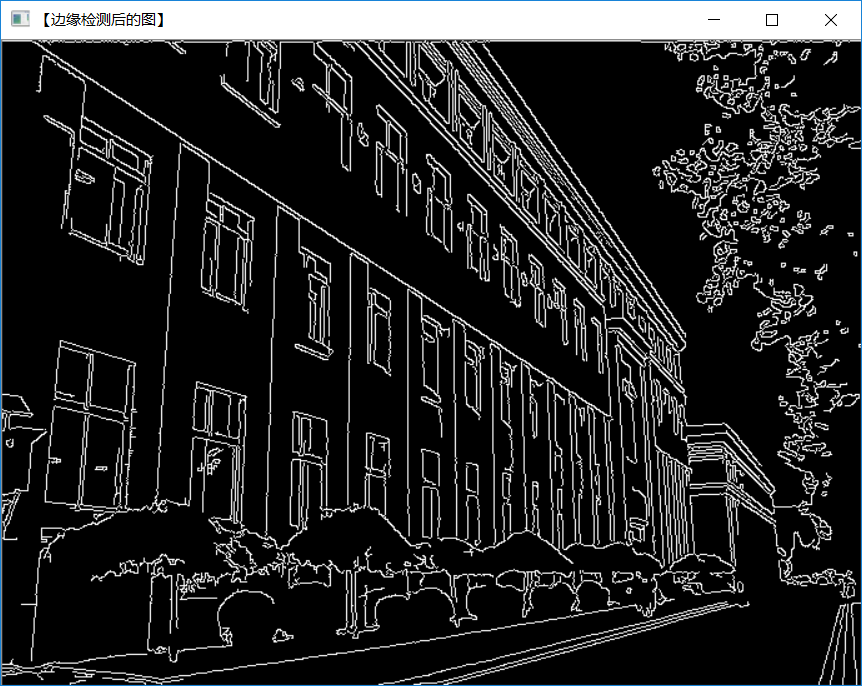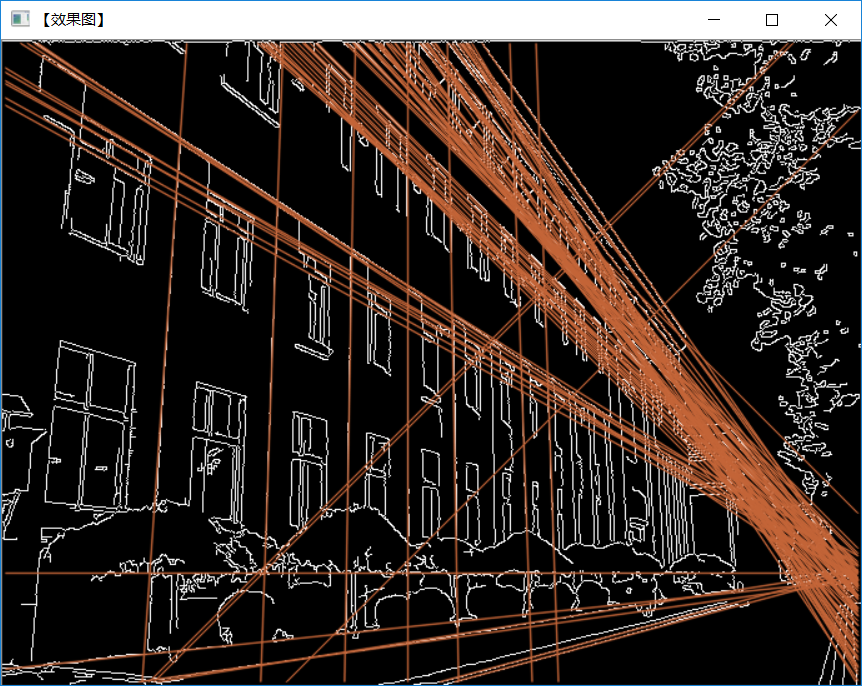# 浅提霍夫变换提取圆

我们可以使用这个相同的程序来检测具有分析描述的其他特征。例如，在圆圈的情况下，参数方程为

(xa)2+(yb)2=r2 ( x − a ) 2 + ( y − b ) 2 = r 2

其中 a a $a$$b$$b$是圆心的坐标并且是 r r $r$半径。在这种情况下，算法的计算复杂度开始增加，因为我们现在在参数空间和三维累加器中有三个坐标。（通常，累加器阵列的计算和大小随着参数数量的增加而多项式增加，因此，基本霍夫技术仅适用于简单曲线。）

## 步骤

它的算法步骤如下：

1. 首先创建累加器空间，由每个像素单元格构成。最初每个单元格都设置为0。
2. 然后对于每个图像中的边缘点$\left(i,j\right)$$(i,j)$，按照圆方程 (ia)2+(jb)2=r2 ( i − a ) 2 + ( j − b ) 2 = r 2 $(i-a)^2+(j-b)2=r^2$将那些可能是一个圆中心的单元格值进行累加。这些单元格在等式中由字母 a a $a$表示。
3. 然后在前面的步骤中由每个可能找到的值$a$$a$，区找到满足等式的所有可能值 b b $b$
4. 搜索累加器空间中的局部最大值。这些单元格表示算法检测到的圆圈。

如果我们不知道事先定位的圆的半径，可以使用三维累加器空间来搜索具有任意半径的圆。当然，这在计算上更加昂贵。

该方法还可以检测部分位于累加器空间外部的圆，只要该圆的区域内仍有足够的圆。

## 霍夫变换提取圆的一些实现链接

matlab关于霍夫变换提取圆
Matlab圆提取

关于opencv的提取圆(给出了C++,C和Python)
Opencv官方文档关于圆提取

python实现提取圆
Python圆提取

# 浅提广义霍夫变换

当我们希望隔离的特征的形状不具有描述其边界的简单解析方程时，使用广义Hough变换。在这种情况下，我们不使用曲线的参数方程，而是使用查找表来定义边界位置和方向与霍夫参数之间的关系。（必须使用原型形状在初步阶段计算查找表值。）

例如，假设我们知道所需特征的形状和方向。（见下图）我们可以$\left({x}_{ref},{y}_{ref}\right)$$(x_{ref},y_{ref})$在特征中指定一个任意参考点，其中定义了特征的形状（即 r r $r$从边界到这个参考点的法线的距离和角度$\omega$$\omega$。我们的查找表（即 R表）将由这些距离和方向对组成，由边界的方向 ω ω $\omega$索引。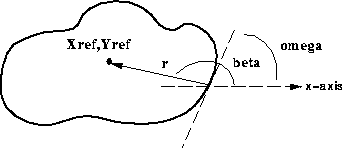霍夫变换空间现在是根据图像中形状的可能位置来定义的，即可能的范围 (xref,yref) ( x r e f , y r e f ) $(x_{ref},y_{ref})$。换句话说，转换定义为：

xref=x+rcos(β)(4) (4) x r e f = x + r cos ⁡ ( β )

yref=y+rsin(β)(5) (5) y r e f = y + r sin ⁡ ( β )

（对于特定的已知方向 r r $r$,$\beta$$\beta$值和来自于表 ω ω $\omega$值）。如果所需特征的方向未知，则该过程变得复杂，因为我们必须通过引入额外参数来扩展累加器，以考虑方向。

# 基本霍夫变换的限制

霍夫变换只有在大量投票落入正确的分箱时才有效，因此可以在背景噪音中轻松检测分箱。这意味着垃圾箱不能太小，否则有些选票会落入邻近垃圾箱，从而降低主垃圾箱的可见度。

另外，当参数数量很大时（也就是说，当我们使用通常超过三个参数的霍夫变换时），单个分箱中投的平均投票数非常低，而这些分箱对应的实际数字在图像中的投票数量并不一定比其邻居多得多。复杂性以一定的速率增加 O(Am2) O ( A m − 2 ) ${\displaystyle{\mathcal {O}} \left({A^{m-2}}\right)}$ ，其中每个附加参数 A A $A$是图像空间的大小和 m m $m$是参数的数量。因此，必须非常小心地使用Hough变换来检测线条或圆圈以外的任何其他内容。

# 结语

本博文主要描述基本经典的霍夫变换，描述了霍夫变换如何提取直线及其原理，展示了部分例子和代码实现，也扩展了一部分霍夫变换的简单描述，希望能对看者有所借鉴。

07-14198
05-253791

07-235935
02-245234
04-081万+
02-179850
05-069万+
08-202万+
07-075033
10-283040
10-31783
07-06739
08-018701
06-24649
09-0359
05-16136

### “相关推荐”对你有帮助么？

•非常没帮助
•没帮助
•一般
•有帮助
•非常有帮助

©️2022 CSDN 皮肤主题：Age of Ai 设计师：meimeiellieYuYunTan

¥2 ¥4 ¥6 ¥10 ¥20余额支付 (余额：-- )扫码支付获取中扫码支付点击重新获取扫码支付1.余额是钱包充值的虚拟货币，按照1:1的比例进行支付金额的抵扣。
2.余额无法直接购买下载，可以购买VIP、C币套餐、付费专栏及课程。余额充值